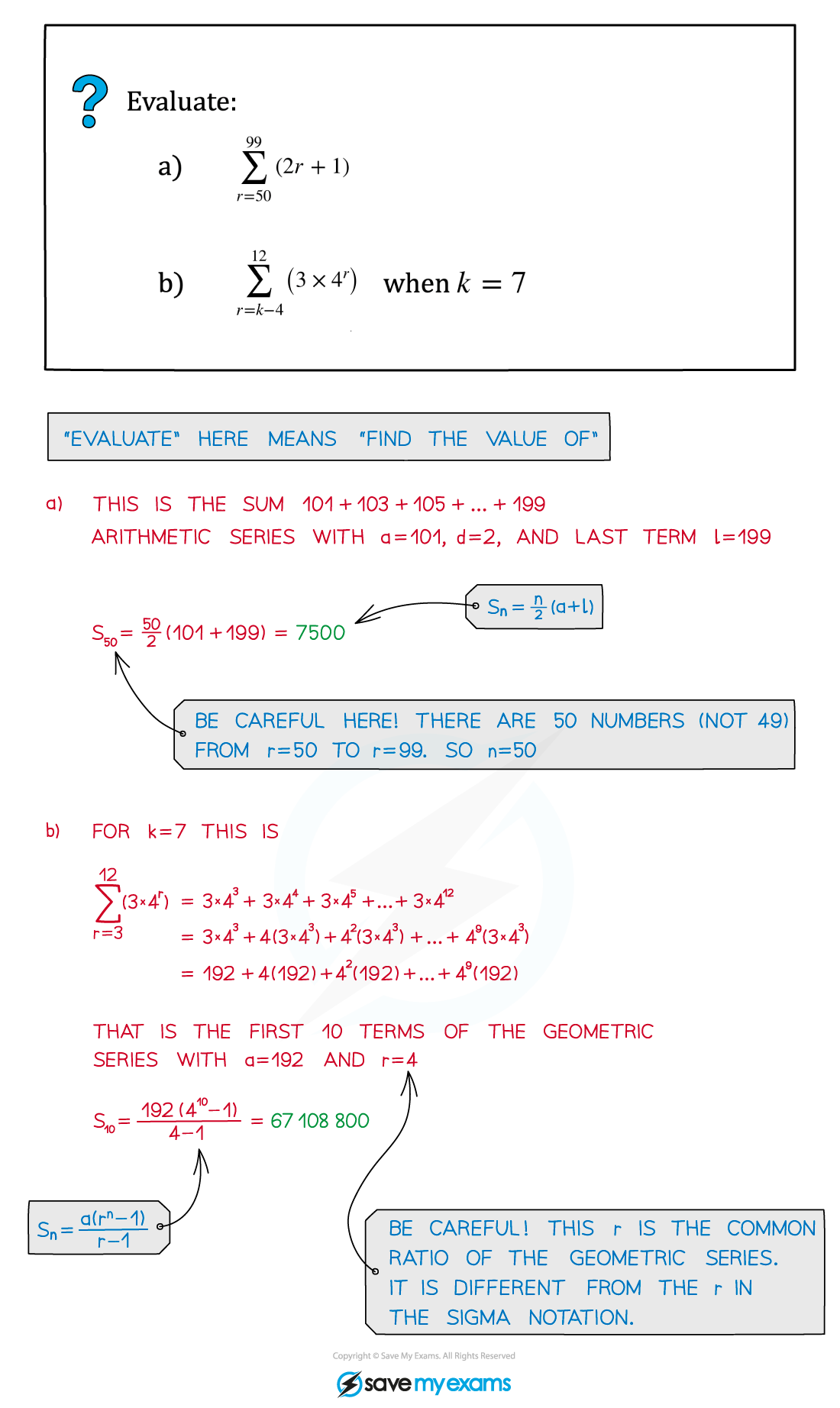# AQA A Level Maths: Pure复习笔记4.5.2 Sigma Notation

### Sigma Notation

#### What is sigma notation?

• The symbol Σ is the capital Greek letter sigma – that's why it's called 'sigma notation'!
• 'Σ' stands for 'sum' – the expression to the right of the Σ tells you what is being summed, and the limits above and below tell you which terms you are summing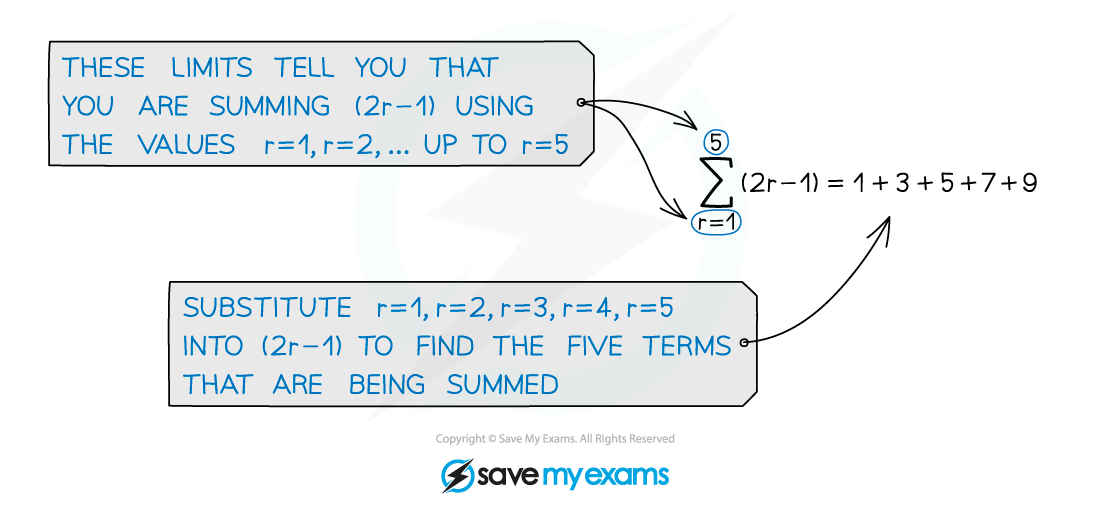• Be careful – the limits don't have to start with 1!

####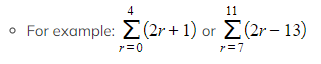What do I need to be able to do with sigma notation?

• Sigma notation can be used to represent both arithmetic series and geometric series
•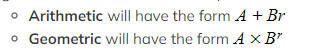Writing out the first few terms will help you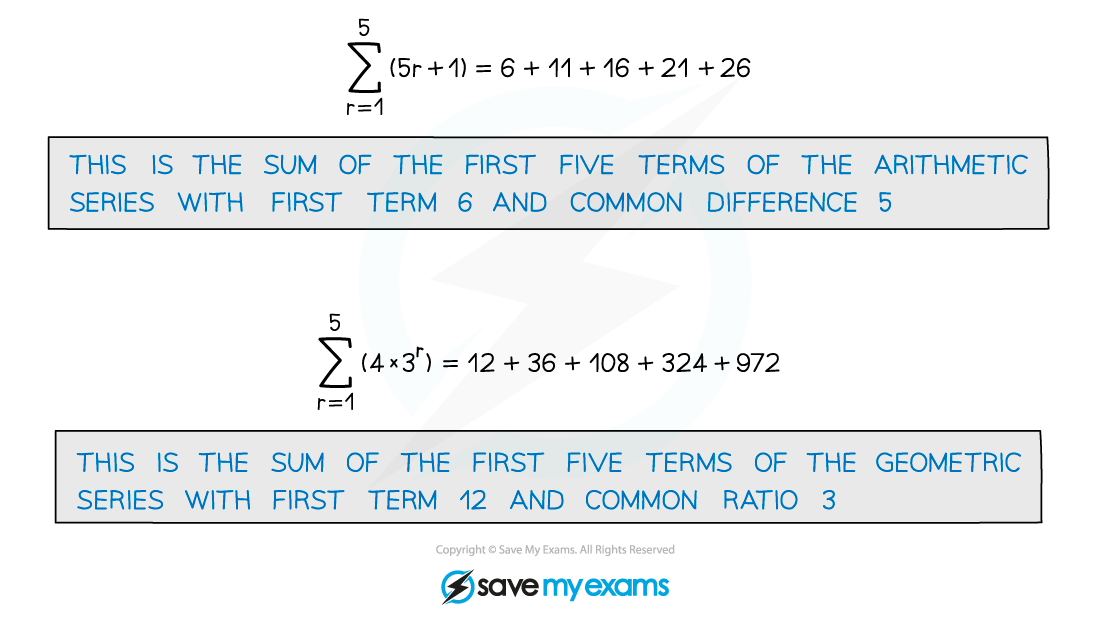• To work out such a sum use the arithmetic and geometric series formulae
• As long as the expressions being summed are the same you can add and subtract in sigma notation
• For example: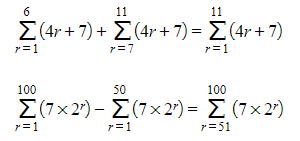#### Exam Tip

Be careful when more than one letter appears in a sigma notation question.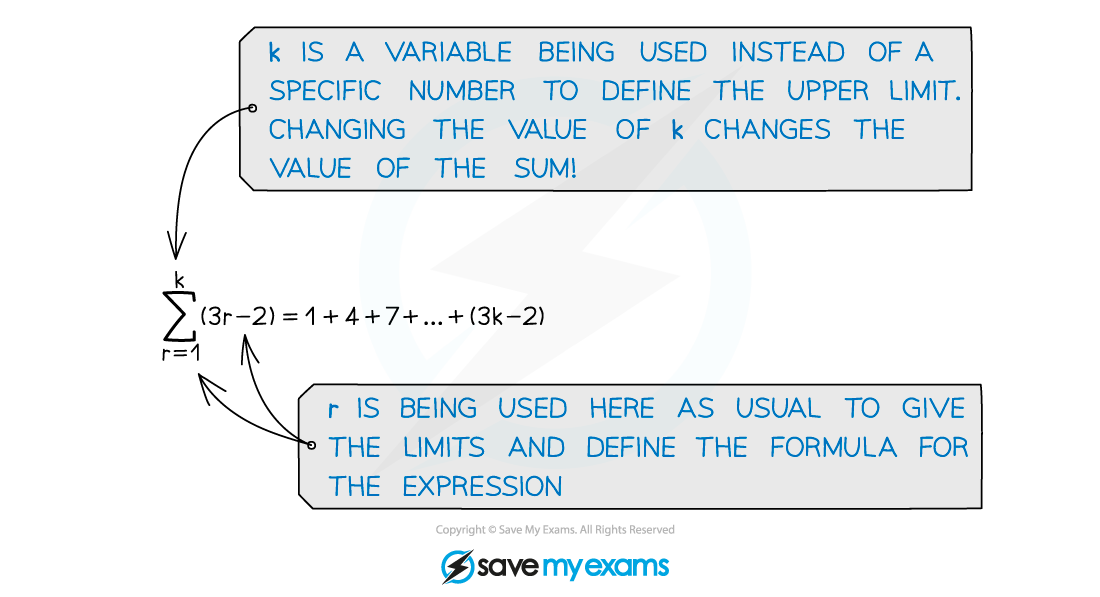#### Worked Example